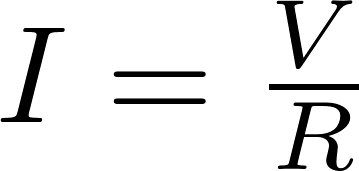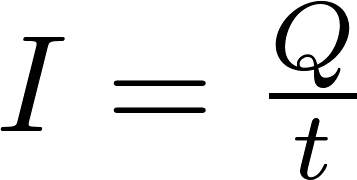# Current

Electric current is the flow of electric charge. This charge is often carried by electrons moving through a wire. The electrons flow from the negative terminal to the positive one, while the current flows from the positive terminal to the negative terminal. The symbol for current is I and current is measured in ampere (A). The current is equal to the charge flowing through a surface divided by the time.

### Ohm's law

Ohm's law

Ohm's law describes the relation between Voltage, Current and Resistance. The law states that the voltage is equal to the current multiplied by the resistance. Ohm's law is named after the German physicist Georg Ohm.

### Formula

The current can be calculated by dividing the voltage by the resistance:The current is equal to the charge divided by the time:R is the symbol for resistance and is measured in ohm (Ω).
V is the symbol for voltage and is measured in volt (V).
I is the symbol for current and is measured in ampere (A).
Q is the symbol for charge and is measured in coulomb (C).
t is the symbol for time and is measured in seconds (s).